#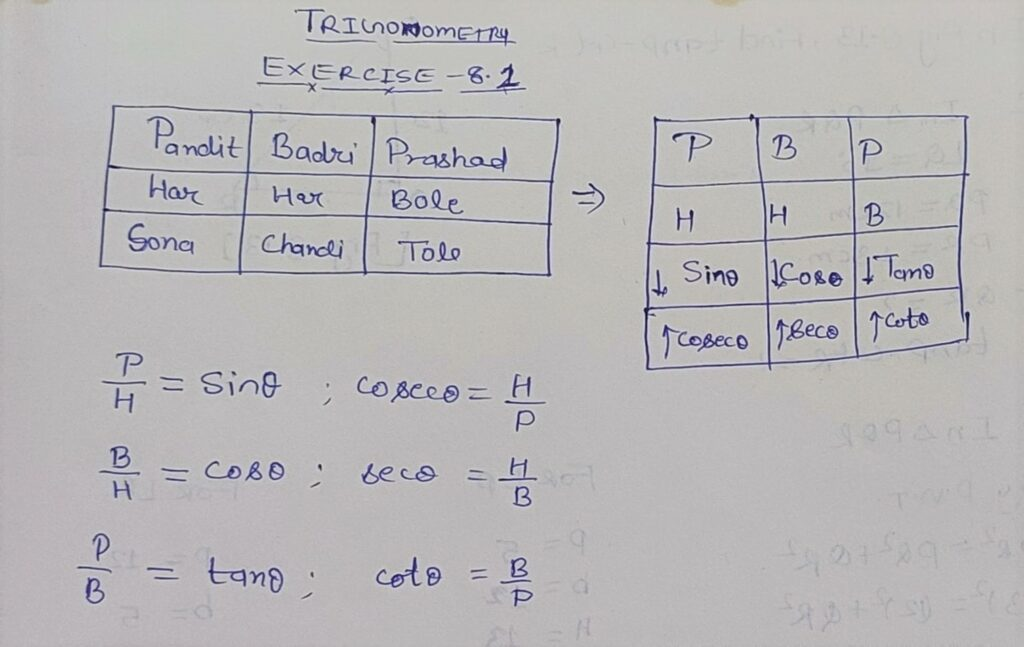Q 1. In ΔABC, right-angled at B, AB = 24cm, BC = 7cm. Determine:

(i) sin A, cos A

(ii) sin C, cos C

Given:

In ΔABC

∠B = 90°

AB = 24

BC = 7

To Find:

(i) sin A =?

Cos A =?

(ii) sin C =?

Cos C =?

Solution: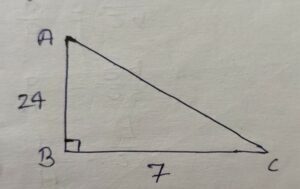By PGT

(AC)2 = (AB)2+(BC )2

=> (AC)2  = (24 )2 + (7 )2

=> (AC)2 = 576 + 49

=> (AC)2 = 625

=> AC = √625

=> AC = 25

(i) For ∠A

P = 24

b = 7

H = 25

(i) sin A = P/H = 24/25

cos A = B/H = 7/25

(ii) For ∠C

P = 7

b = 24

H = 25

sin C = 7/25

cos C = b/H = 24/25

Q 2. In Fig. 8.13,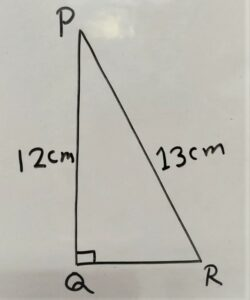find: tan P – cot R

(PR)2 = (PQ)2 + (QR)2

=> (13)2 = (12 )2 + (QR)2

=> 169 = 144 + (QR)2

=> 169 – 144 = (QR)2

=> 25 = (QR)2

=> √25 = QR

=> 5 = QR

For ∠P

P = 5

b = 12

H = 13

tanP = P/H = 5/13

For √ R

P = 12

b = 5

H = 13

cot R = b/H = 5/13

tanP-cotR

= 5/13 – 5/13

= 0

Q3. If sin A = 3/4 , calculate cos A and tan A.

solution:

sin A = 3/4 = P/H

P = 3

H = 4

H = √H2 – P2

=> H = √42– 32

=> H = √16 – 9

=> H = √7

cos A = b/H = 4/√7

tan A = P/b = 3/√7

Q4. Given 15 cot A = 8, Find sin A and sec A

Solution:

15 cot A = 8

cot A = 8/15 = b/P

b = 8

P = 15

H =?

By PGT

H = √P2+b2

=> H = √(15)2 + (8)2

=> H = √225 + 64

=> H = √289

=> H = √17×17

=> H = 17

sin A = P/H = 15/17

sec A = b/H = 8/17

Q5. If sec θ = 13/12, calculate all other trigonometric ratios.

Solution:

sec A = 13/12 = H/b

H = 13

b = 12

P = √H2 – b2

=> P = √132 – 122

=>P = √132 – 122

=>P = √169 – 144

=>P = √25

P = 5

For ∠ θ

P = 5

b = 12

H = 13

sin θ = P/H = 5/13

cos θ = b/H = 12/13

tan θ = P/b = 5/12

cot θ = b/P = 12/5

sec θ = H/b = 13/12

cosec θ = H/P = 13/5

Q6. If ∠A and ∠B are acute angles such that cos A=cos B, then show that ∠A = ∠B.

Given:

∠A < 90º  and

∠B < 90°

cos A = cos B

To Show:

∠A = ∠B

Solution: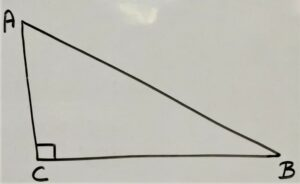In ΔABC

∠C = 90°

For ∠A

P = BC

b = AC

H = AB

Cos A = b/H

=> Cos A = AC/AB    …………………..(i)

For ∠B

P = AC

b = BC

H = AB

Cos B = b/H

=>Cos B = BC/AB     …………………..(ii)

Cos A = Cos B             [ Given   ]

AC/AB = BC/AB        [ From Equation(i) and Equation(ii) ]

=> AC = BC

∴ ΔABC is an isosceles triangle

We know that in isosceles triangle equal sides of opposite angles are also equal

∴   ∠A = ∠B

Q 7. If cot θ = 7/8, evaluate:

(i) (1 + sin θ)(1 – sin θ)/(1 + cos θ ) (1 – cos θ)

(ii) cotθ

Solution:

cot θ = 7/8 = b/P

b = 7

p = 8

H =?

By PGT

H = √P2 + b2

=> H = √82 + 72

=> H = √64 + 49

=> H = √113

sin θ = P/H = 8/√113

cos θ = b/H = 7/√113

(i) (1+sin θ) (1 – sin θ) / (1 + cos θ ) (1 – cos θ)

=> (1 + 8/√113) (1 – 8/√113) / (1 + 7/√113) (1 – 7/√113)

=> [(1)2-(8/√113)2 ] / [ (1)2  –  (7/√113)2 ]

=> [ 1-64/113] / [ 1 – 49 /113 ]

=> [(113 – 64)/113]  /  [ ( 113 – 49/113) ]

=> (47 /  113)   / ( 64/113)

=> 47/64      ans

(ii) cot2θ = (7/8)2 = 49/64

Q8). If 3 cot A = 4, check whether 1 – tan2 A  / 1 + tan2A = cos2 A – sin2 A or not.

Given:

3 cot A = 4

To Check:

1 – tan2 / 1 + tan2A  = cos2 A – sin2 A or not

Solution:

3 cot A = 4

=> cot A = 4/3 = b/P

b = 4

P = 3

H =?

H = √32 + 42

=> H = √ (9 + 16)

=> H = √25

=> H = 5

sin A = P/H = 3/5

cos A = b/H = 4/5

tan A = P/B = 3/4

1 – tan2 / 1 + tan2 A  = cos2 A – sin2A

L.H.S. = 1 – tan2/ 1 + tan2A

= 1 – (3/4)2  / 1 + (3/4)2

= [1 – 9/16 ]/ [1 + 9/16]

= [ (16 – 9) /16 ] / [ (16 + 9) /16 ]

= [ (7)/16] / [ (25)/16 ]

= 7/25

R.H.S. = cos2 A – sin2A

=> (4/5)2 – (3/5)2

=> 16/25 – 9/25

=> (16-9) / 25

=> 7/25

L.H.S. = R.H.S.                         ( Hance Proved )

Q.9) In triangle ABC, right-angled at B, if tan A = 1/√3, find the value of:

(i)sin A cos C + cos A sin C

(ii) cos A cos C – sin A sin C

Given:In Δ ABC

∠B = 90º

tan A = 1/√3

To Find:

(i) sin A cos C + cos A sin C

(ii) cos A cos C – sin A sin C

Solution:

tan A = 1/√3 = P/H

P = 1

b = √3

H2 = P 2 + b 2

H2 = (1 )2 + (√3)2

=> H2 = (1 )2 + (√3)2

=> H2 = 1 + 3

=> H2 = 4

=> H = 2

For ∠A

P = 1

b = √3

H = 2

sin A = P/H = 1/2

cos A = b/H = √3/2

For ∠C

P = √3

b = 1

H = 2

sin C = P/H = √3/2

cos C = b/H = 1/2

(i) sin A cos C + cos A sin C

= 1/2×1/2 + √3/2 × √3/2

= 1/4 + 3/4

= 4/4

= 1

(ii) cos A cos C – sin A sin C

= √3/2×1/2 – 1/2 × √3/2

= √3/4 – √3/4

= 0

Q.10) In ΔPQR, right-angled at Q, PR + QR = 25cm and PQ = 5cm. Determine the values of sin P, Cos P, and tan P.

Given:

In ΔPQR

∠Q = 90º

PR + QR = 25cm

PQ = 5cm

To Find:

sin p =?

cos p =?

tan p =?

Solution:

According to figure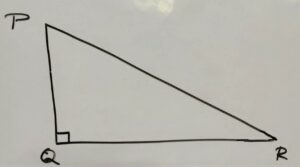P = 5cm

PR + QR = 25cm

=>H+b = 25

=>H = 25-b     ……………(i)

H2 = p2+b2

=> (25-b)2 = 52+b2

=> (25)2 – 2×25×b + b2 = 25 + b2

=> 625 – 50b + b2 = 25 + b2

=> – 50b + b2 – b2 = 25 – 625

=> – 50b = – 600

=> b = – 600/- 50

=> b = 12 Put into equation number (i)

H = 25 – b     ……………(i)

=> H = 25 – 12

=>H = 13

now For ∠p

p = 5

b = 12

H = 13

sin P = P/H = 5/13

cos P = b/H = 12/13

tan P = P/b = 5/12

Q.11) State whether the following are true or false. justify your answer.

(i) The value of tan A is always less than 1.

Ans: False

justification:

(ii) sec A = 12/5 for some value of angle A.

Ans: True

(iii) cos A is the abbreviation used for the cosecant of angle A.

Ans: True

(iv) cot A is the product of cot and A

Ans: False

(v) sin θ = 4/3 for some angle θ.

Ans: False